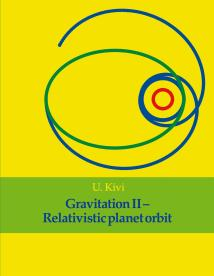# Kuopion kaupunginkirjastoIsbn: 978-95-2805-734-5
Publisher: Books on Demand
Category:

Accessible since: April 2021

E-book

## Gravitation II: Relativistic planet orbit

Values for the integration constants in the relativistic orbit equation are constructed in this book as functions of Schwarzschild radius and the planet maximum and minimum distances from the Sun. An algorithm to calculate the planet orbit according to relativity theory is presented in the book. A computer program that uses the algorithm has been listed in an appendix. Eight different cases have been calculated; the sun or black hole mass has been varied in these cases. The orbit graphs are presented in figures. The perihelion shift is presented as a function of the polar angle coordinate in every suitable calculation case. The text has been clarified with more than 20 graphs describing the form of the planet orbit.